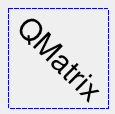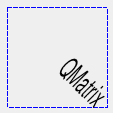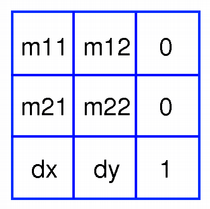/Qt 5.13

# QMatrix Class

The QMatrix class specifies 2D transformations of a coordinate system. More...

 Header: #include qmake: QT += gui

This class is obsolete. It is provided to keep old source code working. We strongly advise against using it in new code.

## Public Functions

 QMatrix(const QMatrix &matrix) QMatrix(qreal m11, qreal m12, qreal m21, qreal m22, qreal dx, qreal dy) QMatrix() QMatrix & operator=(const QMatrix &matrix) qreal m11() const qreal m12() const qreal m21() const qreal m22() const qreal determinant() const qreal dx() const qreal dy() const QMatrix inverted(bool *invertible = nullptr) const bool isIdentity() const bool isInvertible() const void map(qreal x, qreal y, qreal *tx, qreal *ty) const void map(int x, int y, int *tx, int *ty) const QPoint map(const QPoint &point) const QPointF map(const QPointF &point) const QLine map(const QLine &line) const QLineF map(const QLineF &line) const QPolygonF map(const QPolygonF &polygon) const QPolygon map(const QPolygon &polygon) const QRegion map(const QRegion ®ion) const QPainterPath map(const QPainterPath &path) const QRectF mapRect(const QRectF &rectangle) const QRect mapRect(const QRect &rectangle) const QPolygon mapToPolygon(const QRect &rectangle) const void reset() QMatrix & rotate(qreal degrees) QMatrix & scale(qreal sx, qreal sy) void setMatrix(qreal m11, qreal m12, qreal m21, qreal m22, qreal dx, qreal dy) QMatrix & shear(qreal sh, qreal sv) QMatrix & translate(qreal dx, qreal dy) QVariant operator QVariant() const bool operator!=(const QMatrix &matrix) const QMatrix operator*(const QMatrix &matrix) const QMatrix & operator*=(const QMatrix &matrix) bool operator==(const QMatrix &matrix) const
 bool qFuzzyCompare(const QMatrix &m1, const QMatrix &m2) uint qHash(const QMatrix &key, uint seed = 0) QPoint operator*(const QPoint &point, const QMatrix &matrix) QPointF operator*(const QPointF &point, const QMatrix &matrix) QLineF operator*(const QLineF &line, const QMatrix &matrix) QLine operator*(const QLine &line, const QMatrix &matrix) QPolygon operator*(const QPolygon &polygon, const QMatrix &matrix) QPolygonF operator*(const QPolygonF &polygon, const QMatrix &matrix) QRegion operator*(const QRegion ®ion, const QMatrix &matrix) QPainterPath operator*(const QPainterPath &path, const QMatrix &matrix) QDataStream & operator<<(QDataStream &stream, const QMatrix &matrix) QDataStream & operator>>(QDataStream &stream, QMatrix &matrix)

## Detailed Description

A matrix specifies how to translate, scale, shear or rotate the coordinate system, and is typically used when rendering graphics. QMatrix, in contrast to QTransform, does not allow perspective transformations. QTransform is the recommended transformation class in Qt.

A QMatrix object can be built using the setMatrix(), scale(), rotate(), translate() and shear() functions. Alternatively, it can be built by applying basic matrix operations. The matrix can also be defined when constructed, and it can be reset to the identity matrix (the default) using the reset() function.

The QMatrix class supports mapping of graphic primitives: A given point, line, polygon, region, or painter path can be mapped to the coordinate system defined by this matrix using the map() function. In case of a rectangle, its coordinates can be transformed using the mapRect() function. A rectangle can also be transformed into a polygon (mapped to the coordinate system defined by this matrix), using the mapToPolygon() function.

QMatrix provides the isIdentity() function which returns `true` if the matrix is the identity matrix, and the isInvertible() function which returns `true` if the matrix is non-singular (i.e. AB = BA = I). The inverted() function returns an inverted copy of this matrix if it is invertible (otherwise it returns the identity matrix). In addition, QMatrix provides the determinant() function returning the matrix's determinant.

Finally, the QMatrix class supports matrix multiplication, and objects of the class can be streamed as well as compared.

### Rendering Graphics

When rendering graphics, the matrix defines the transformations but the actual transformation is performed by the drawing routines in QPainter.

By default, QPainter operates on the associated device's own coordinate system. The standard coordinate system of a QPaintDevice has its origin located at the top-left position. The x values increase to the right; y values increase downward. For a complete description, see the coordinate system documentation.

QPainter has functions to translate, scale, shear and rotate the coordinate system without using a QMatrix. For example:```void SimpleTransformation::paintEvent(QPaintEvent *) { QPainter painter(this); painter.setPen(QPen(Qt::blue, 1, Qt::DashLine)); painter.drawRect(0, 0, 100, 100); painter.rotate(45); painter.setFont(QFont("Helvetica", 24)); painter.setPen(QPen(Qt::black, 1)); painter.drawText(20, 10, "QMatrix"); }```

Although these functions are very convenient, it can be more efficient to build a QMatrix and call QPainter::setMatrix() if you want to perform more than a single transform operation. For example:```void CombinedTransformation::paintEvent(QPaintEvent *) { QPainter painter(this); painter.setPen(QPen(Qt::blue, 1, Qt::DashLine)); painter.drawRect(0, 0, 100, 100); QMatrix matrix; matrix.translate(50, 50); matrix.rotate(45); matrix.scale(0.5, 1.0); painter.setMatrix(matrix); painter.setFont(QFont("Helvetica", 24)); painter.setPen(QPen(Qt::black, 1)); painter.drawText(20, 10, "QMatrix"); }```

### Basic Matrix OperationsA QMatrix object contains a 3 x 3 matrix. The `dx` and `dy` elements specify horizontal and vertical translation. The `m11` and `m22` elements specify horizontal and vertical scaling. And finally, the `m21` and `m12` elements specify horizontal and vertical shearing.

QMatrix transforms a point in the plane to another point using the following formulas:

```x' = m11*x + m21*y + dx
y' = m22*y + m12*x + dy```

The point (x, y) is the original point, and (x', y') is the transformed point. (x', y') can be transformed back to (x, y) by performing the same operation on the inverted() matrix.

The various matrix elements can be set when constructing the matrix, or by using the setMatrix() function later on. They can also be manipulated using the translate(), rotate(), scale() and shear() convenience functions, The currently set values can be retrieved using the m11(), m12(), m21(), m22(), dx() and dy() functions.

Translation is the simplest transformation. Setting `dx` and `dy` will move the coordinate system `dx` units along the X axis and `dy` units along the Y axis. Scaling can be done by setting `m11` and `m22`. For example, setting `m11` to 2 and `m22` to 1.5 will double the height and increase the width by 50%. The identity matrix has `m11` and `m22` set to 1 (all others are set to 0) mapping a point to itself. Shearing is controlled by `m12` and `m21`. Setting these elements to values different from zero will twist the coordinate system. Rotation is achieved by carefully setting both the shearing factors and the scaling factors.

Here's the combined transformations example using basic matrix operations:```void BasicOperations::paintEvent(QPaintEvent *) { double pi = 3.14; double a = pi/180 * 45.0; double sina = sin(a); double cosa = cos(a); QMatrix translationMatrix(1, 0, 0, 1, 50.0, 50.0); QMatrix rotationMatrix(cosa, sina, -sina, cosa, 0, 0); QMatrix scalingMatrix(0.5, 0, 0, 1.0, 0, 0); QMatrix matrix; matrix = scalingMatrix * rotationMatrix * translationMatrix; QPainter painter(this); painter.setPen(QPen(Qt::blue, 1, Qt::DashLine)); painter.drawRect(0, 0, 100, 100); painter.setMatrix(matrix); painter.setFont(QFont("Helvetica", 24)); painter.setPen(QPen(Qt::black, 1)); painter.drawText(20, 10, "QMatrix"); }```

## Member Function Documentation

### QMatrix::QMatrix(const QMatrix &matrix)

Constructs a matrix that is a copy of the given matrix.

### QMatrix::QMatrix(qrealm11, qrealm12, qrealm21, qrealm22, qrealdx, qrealdy)

Constructs a matrix with the elements, m11, m12, m21, m22, dx and dy.

### QMatrix::QMatrix()

Constructs an identity matrix.

All elements are set to zero except `m11` and `m22` (specifying the scale), which are set to 1.

### QMatrix &QMatrix::operator=(const QMatrix &matrix)

Assigns the given matrix's values to this matrix.

### qreal QMatrix::m11() const

Returns the horizontal scaling factor.

### qreal QMatrix::m12() const

Returns the vertical shearing factor.

### qreal QMatrix::m21() const

Returns the horizontal shearing factor.

### qreal QMatrix::m22() const

Returns the vertical scaling factor.

### qreal QMatrix::determinant() const

Returns the matrix's determinant.

This function was introduced in Qt 4.6.

### qreal QMatrix::dx() const

Returns the horizontal translation factor.

### qreal QMatrix::dy() const

Returns the vertical translation factor.

### QMatrix QMatrix::inverted(bool *invertible = nullptr) const

Returns an inverted copy of this matrix.

If the matrix is singular (not invertible), the returned matrix is the identity matrix. If invertible is valid (i.e. not 0), its value is set to true if the matrix is invertible, otherwise it is set to false.

### bool QMatrix::isIdentity() const

Returns `true` if the matrix is the identity matrix, otherwise returns `false`.

### bool QMatrix::isInvertible() const

Returns `true` if the matrix is invertible, otherwise returns `false`.

### void QMatrix::map(qrealx, qrealy, qreal *tx, qreal *ty) const

Maps the given coordinates x and y into the coordinate system defined by this matrix. The resulting values are put in *tx and *ty, respectively.

The coordinates are transformed using the following formulas:

```x' = m11*x + m21*y + dx
y' = m22*y + m12*x + dy```

The point (x, y) is the original point, and (x', y') is the transformed point.

### void QMatrix::map(intx, inty, int *tx, int *ty) const

Maps the given coordinates x and y into the coordinate system defined by this matrix. The resulting values are put in *tx and *ty, respectively. Note that the transformed coordinates are rounded to the nearest integer.

### QPoint QMatrix::map(const QPoint &point) const

Creates and returns a QPoint object that is a copy of the given point, mapped into the coordinate system defined by this matrix. Note that the transformed coordinates are rounded to the nearest integer.

### QPointF QMatrix::map(const QPointF &point) const

Creates and returns a QPointF object that is a copy of the given point, mapped into the coordinate system defined by this matrix.

### QLine QMatrix::map(const QLine &line) const

Creates and returns a QLine object that is a copy of the given line, mapped into the coordinate system defined by this matrix. Note that the transformed coordinates are rounded to the nearest integer.

### QLineF QMatrix::map(const QLineF &line) const

Creates and returns a QLineF object that is a copy of the given line, mapped into the coordinate system defined by this matrix.

### QPolygonF QMatrix::map(const QPolygonF &polygon) const

Creates and returns a QPolygonF object that is a copy of the given polygon, mapped into the coordinate system defined by this matrix.

### QPolygon QMatrix::map(const QPolygon &polygon) const

Creates and returns a QPolygon object that is a copy of the given polygon, mapped into the coordinate system defined by this matrix. Note that the transformed coordinates are rounded to the nearest integer.

### QRegion QMatrix::map(const QRegion &region) const

Creates and returns a QRegion object that is a copy of the given region, mapped into the coordinate system defined by this matrix.

Calling this method can be rather expensive if rotations or shearing are used.

### QPainterPath QMatrix::map(const QPainterPath &path) const

Creates and returns a QPainterPath object that is a copy of the given path, mapped into the coordinate system defined by this matrix.

### QRectF QMatrix::mapRect(const QRectF &rectangle) const

Creates and returns a QRectF object that is a copy of the given rectangle, mapped into the coordinate system defined by this matrix.

The rectangle's coordinates are transformed using the following formulas:

```x' = m11*x + m21*y + dx
y' = m22*y + m12*x + dy```

If rotation or shearing has been specified, this function returns the bounding rectangle. To retrieve the exact region the given rectangle maps to, use the mapToPolygon() function instead.

### QRect QMatrix::mapRect(const QRect &rectangle) const

Creates and returns a QRect object that is a copy of the given rectangle, mapped into the coordinate system defined by this matrix. Note that the transformed coordinates are rounded to the nearest integer.

### QPolygon QMatrix::mapToPolygon(const QRect &rectangle) const

Creates and returns a QPolygon representation of the given rectangle, mapped into the coordinate system defined by this matrix.

The rectangle's coordinates are transformed using the following formulas:

```x' = m11*x + m21*y + dx
y' = m22*y + m12*x + dy```

Polygons and rectangles behave slightly differently when transformed (due to integer rounding), so `matrix.map(QPolygon(rectangle))` is not always the same as `matrix.mapToPolygon(rectangle)`.

### void QMatrix::reset()

Resets the matrix to an identity matrix, i.e. all elements are set to zero, except `m11` and `m22` (specifying the scale) which are set to 1.

### QMatrix &QMatrix::rotate(qrealdegrees)

Rotates the coordinate system the given degrees counterclockwise.

Note that if you apply a QMatrix to a point defined in widget coordinates, the direction of the rotation will be clockwise because the y-axis points downwards.

Returns a reference to the matrix.

### QMatrix &QMatrix::scale(qrealsx, qrealsy)

Scales the coordinate system by sx horizontally and sy vertically, and returns a reference to the matrix.

### void QMatrix::setMatrix(qrealm11, qrealm12, qrealm21, qrealm22, qrealdx, qrealdy)

Sets the matrix elements to the specified values, m11, m12, m21, m22, dx and dy.

Note that this function replaces the previous values. QMatrix provide the translate(), rotate(), scale() and shear() convenience functions to manipulate the various matrix elements based on the currently defined coordinate system.

### QMatrix &QMatrix::shear(qrealsh, qrealsv)

Shears the coordinate system by sh horizontally and sv vertically, and returns a reference to the matrix.

### QMatrix &QMatrix::translate(qrealdx, qrealdy)

Moves the coordinate system dx along the x axis and dy along the y axis, and returns a reference to the matrix.

### QVariant QMatrix::operator QVariant() const

Returns the matrix as a QVariant.

This function was introduced in Qt 4.2.

### bool QMatrix::operator!=(const QMatrix &matrix) const

Returns `true` if this matrix is not equal to the given matrix, otherwise returns `false`.

### QMatrix QMatrix::operator*(const QMatrix &matrix) const

Returns the result of multiplying this matrix by the given matrix.

Note that matrix multiplication is not commutative, i.e. a*b != b*a.

### QMatrix &QMatrix::operator*=(const QMatrix &matrix)

Returns the result of multiplying this matrix by the given matrix.

### bool QMatrix::operator==(const QMatrix &matrix) const

Returns `true` if this matrix is equal to the given matrix, otherwise returns `false`.

## Related Non-Members

### bool QMatrix::qFuzzyCompare(const QMatrix &m1, const QMatrix &m2)

The qFuzzyCompare function is for comparing two matrices using a fuzziness factor.

Returns `true` if m1 and m2 are equal, allowing for a small fuzziness factor for floating-point comparisons; false otherwise.

This function was introduced in Qt 4.6.

### uint QMatrix::qHash(const QMatrix &key, uintseed = 0)

Returns the hash value for key, using seed to seed the calculation.

This function was introduced in Qt 5.6.

### QPoint QMatrix::operator*(const QPoint &point, const QMatrix &matrix)

This is the same as matrix.map(point).

### QPointF QMatrix::operator*(const QPointF &point, const QMatrix &matrix)

Same as matrix.map(point).

### QLineF QMatrix::operator*(const QLineF &line, const QMatrix &matrix)

This is the same as matrix.map(line).

### QLine QMatrix::operator*(const QLine &line, const QMatrix &matrix)

This is the same as matrix.map(line).

### QPolygon QMatrix::operator*(const QPolygon &polygon, const QMatrix &matrix)

This is the same as matrix.map(polygon).

### QPolygonF QMatrix::operator*(const QPolygonF &polygon, const QMatrix &matrix)

This is the same as matrix.map(polygon).

### QRegion QMatrix::operator*(const QRegion &region, const QMatrix &matrix)

This is the same as matrix.map(region).

### QPainterPath QMatrix::operator*(const QPainterPath &path, const QMatrix &matrix)

This is the same as matrix.map(path).

### QDataStream &QMatrix::operator<<(QDataStream &stream, const QMatrix &matrix)

Writes the given matrix to the given stream and returns a reference to the stream.